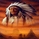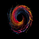2353 visningar
This script was inspired by @ChartArt.
GREEN = UPTREND
RED = DOWNTREND

TREND IS DETERMINED BY IF THE CLOSE IS BELOW OR ABOVE THE SMA SET (DEFAULT 20)
It has the trend of the following time-frames:
1 HR
2 HR
3 HR
4 HR
6 HR
8 HR
12 HR
1 DAY
Open-source script

In true TradingView spirit, the author of this script has published it open-source, so traders can understand and verify it. Cheers to the author! You may use it for free, but reuse of this code in a publication is governed by House Rules. You can favorite it to use it on a chart.

Want to use this script on a chart?
```study(title="TUX Time Trend", shorttitle="TUX Time Trend", overlay=true)
len = input(20, minval=1, title="Length")
src = input(close, title="Source")
out = sma(src, len)
//plot(out, color=blue, title="MA")
s1 = security(ticker, "60", sma(close,len)) // 1 HR
s1c = iff(security(ticker, "60", sma(close,len)) > security(ticker, "60", close), red, green)
plot(s1,linewidth=2, title="1 HR", color=s1c)

s2 = security(ticker, "120", sma(close,len)) // 2 HR
s2c = iff(security(ticker, "120", sma(close,len)) > security(ticker, "120", close), red, green)
plot(s2,linewidth=2, title="2 HR", color=s2c)

s3 = security(ticker, "180", sma(close,len)) // 3 HR
s3c = iff(security(ticker, "180", sma(close,len)) > security(ticker, "180", close), red, green)
plot(s3,linewidth=2, title="3 HR", color=s3c)

s4 = security(ticker, "240", sma(close,len)) // 4 HR
s4c = iff(security(ticker, "240", sma(close,len)) > security(ticker, "240", close), red, green)
plot(s4,linewidth=2, title="4 HR", color=s4c)

s6 = security(ticker, "360", sma(close,len)) // 6 HR
s6c = iff(security(ticker, "360", sma(close,len)) > security(ticker, "360", close), red, green)
plot(s6,linewidth=2, title="6 HR", color=s6c)

s8 = security(ticker, "480", sma(close,len)) // 8 HR
s8c = iff(security(ticker, "480", sma(close,len)) > security(ticker, "480", close), red, green)
plot(s8,linewidth=2, title="8 HR", color=s8c)

s12 = security(ticker, "720", sma(close,len)) // 12 HR
s12c = iff(security(ticker, "720", sma(close,len)) > security(ticker, "720", close), red, green)
plot(s12,linewidth=2, title="12 HR", color=s12c)

sd = security(ticker, "D", sma(close,len)) // D
sdc = iff(security(ticker, "D", sma(close,len)) > security(ticker, "D", close), red, green)
plot(sd,linewidth=2, title="D", color=sdc)

sw = security(ticker, "W", sma(close,len)) // D
swc = iff(security(ticker, "W", sma(close,len)) > security(ticker, "W", close), red, green)
//plot(sw,linewidth=2, title="W", color=swc)

sm = security(ticker, "M", sma(close,len)) // D
smc = iff(security(ticker, "M", sma(close,len)) > security(ticker, "M", close), red, green)
//plot(sm,linewidth=2, title="M", color=smc)

```

## KommentarerHi friends!
The indicator repaints?
I've changed the style in culumns and the signal are very good for entry to the market.
Thanks
SvaraThank you for coding this multi-time-frame trend script!
Svara